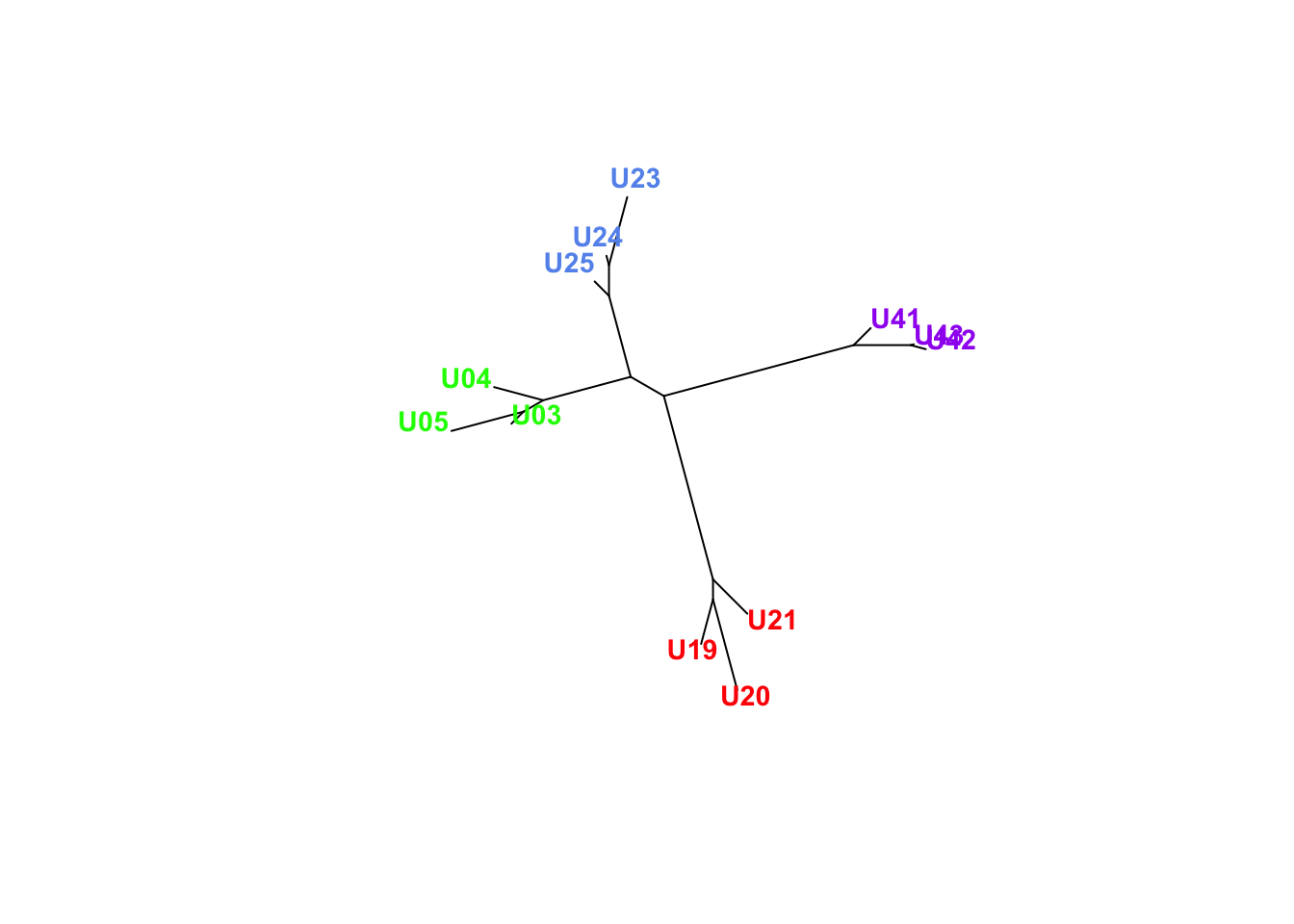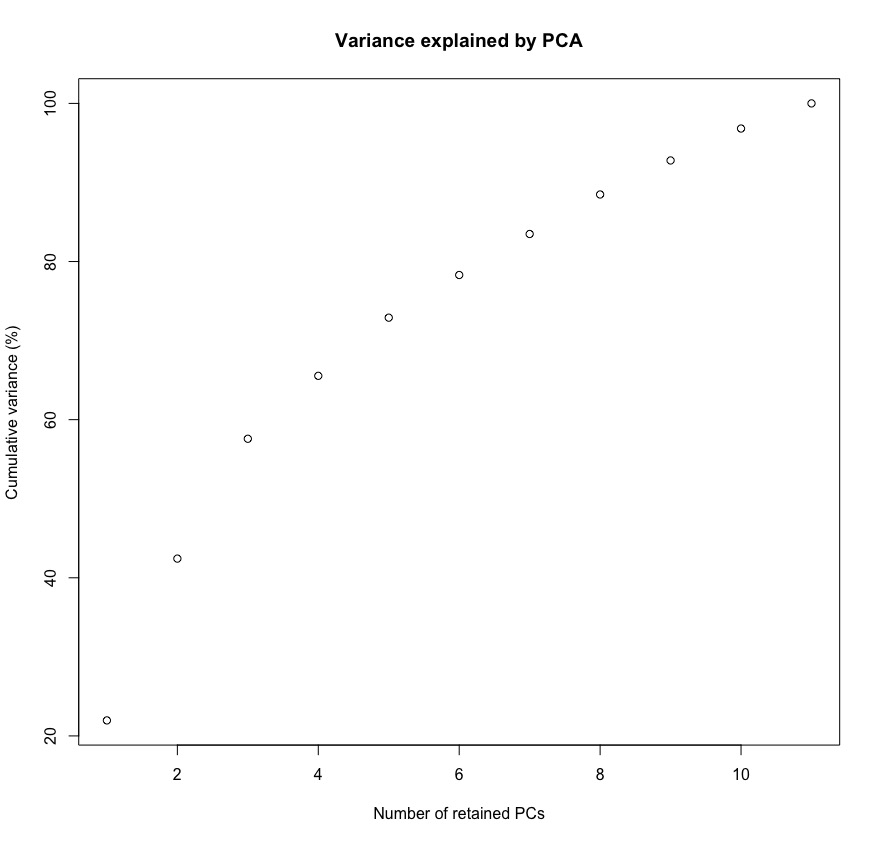Note: This tutorial is written to teach some basic population genomic analyses for NRES 721. We’ll do these exercises entirely in `R`, so folks should be able to follow along regardless of their operating system. Students, you can download all of the data we’ll use by using the `svn checkout https://github.com/twpierson/nres721_popgen_phylo/trunk/data` command in your working directory, from the `/data` directory in the Github repository here, or from Dropbox by clicking here.

## Introduction

In the last tutorial, we assembled a RADseq-style (RADcap) dataset from the patch-nosed salamander (Urspelerpes brucei) in `ipyrad`. We ended up with a total of 884 loci (and 1110 SNPs) in our de novo assembly and 761 loci (and 1755 SNPs) in our reference-based assembly. As you’ve discussed earlier in the semester, there are many inferences you can make from these population genetic data (and a multitude of methods for doing so!). Today, we’ll apply some of the methods you’ve already learned and discuss how large datasets—and in 2019, several hundred loci barely qualifies as “large”!—may present unique challenges. To do this, we’ll work entirely in `R`.

First, let’s load the packages you need. If you don’t already have these installed, do that first.

``````library(phangorn)
library(hierfstat)
library(scales)``````

Then, set your working directory. This should be the folder in which you have the `\data` directory we downloaded earlier.

``setwd("[your working directory]")``

## Neighbor-joining tree

For an easy, preliminary visualization of our data, let’s build a neighbor-joining tree. This will (very quickly) estimate a topology from a distance matrix, which we’ll calculate from our SNP data. There are many good reasons not to ultimately read too much into this topology, but it can provide a good jumping-off point to check our data.

First, we’ll read in one of the output files from the `ipyrad` de novo assembly. The file extension `usnps` tells us that this is a PHYLIP file consisting of one random SNP per locus.

``ubrucei_phydat <- read.phyDat("data/ubrucei_denovo.usnps", format="phy", type="DNA")``

Discussion: Why might we only include one SNP per locus?

Let’s examine this object we’ve just created.

``ubrucei_phydat``
``````## 12 sequences with 396 character and 385 different site patterns.
## The states are a c g t``````

Discussion: This suggests that we have 396 “character patterns”. What does that mean? Why is this number smaller than the number of loci we have?

From these data, we’ll first create a distance matrix.

``ubrucei_dm <- dist.ml(ubrucei_phydat, exclude="pairwise")``

From this distance matrix, we’ll create a neighbor-joining tree.

``ubrucei_NJtree <- NJ(ubrucei_dm)``

And we’ll now plot that as an unrooted tree, coloring the tips to match the collection locality of each sample.

``````plot.phylo(ubrucei_NJtree, cex=0.9, type='u',
tip.col = c(rep("green",3), rep("red",3), rep("cornflowerblue",3), rep("purple",3)),
font = 2, x.lim = c(-0.1, 0.4))``````Discussion: Just examining this visually, what do you notice? Does this serve as a good “sanity check” for our data?

## DAPC

This neighbor-joining tree provides a hint that our collection localities may be genetically differentiated, but it’s a very qualitative evaluation. Next, we’ll try a method to estimate how many “populations” or “clusters” actually exist among these data. (Of course, understanding the biological meaning of a “population” or “cluster” here might also involve some critical thinking!)

There are a variety of programs—with a diversity of underlying philosophies and statistical methods—to conduct this kind of analysis. For example, you used `Structure` in an earlier class. Today, we’ll try a discriminant analysis of principal components (DAPC). This method estimates the number of “genetic clusters” in the data and then estimates probabilistic individual assignments to each cluster. For a much more detailed description of these analyses and a tutorial of how to conduct them, see here.

For this analysis, let’s read in a different output file from `ipyrad`. The file extension (`.str`) tells us that this is a `Structure`-formatted output file, and it contains data for all variable sites. We need to provide the `read.structure()` function a few more pieces of information (e.g., number of individuals, number of loci), which we can glean from the summary statistics file we viewed in the last tutorial.

``````ubrucei_strdat <- read.structure(file="data/ubrucei_denovo.str",
n.ind = 12, n.loc = 1110,
onerowperind = FALSE, col.lab=1,
col.pop=0, NA.char="-9",col.other=0,
row.marknames=0)``````

The first step of the DAPC is to estimate the number of clusters. We’ll use the `find.clusters()` function to do this, and it’ll display interactive plots to help use choose the numbers of PCs to retain and the number of clusters. We’ll also set the seed to make sure this example is reproducible among all of us.

``````set.seed(1234)
ubrucei_clust <- find.clusters(ubrucei_strdat, max.n.clust = 5)``````This plot shows the cumulative variance explained by including progressively more principal components. It looks like there’s really no plateau of cumulative variance, so we can retain all 11 PCs. Enter “11” into the prompt.